### EXP Function – Exponential Value in Excel, VBA, Goggle Sheets

This tutorial demonstrates how to use the Excel EXP Function in Excel to calculate the exponential value.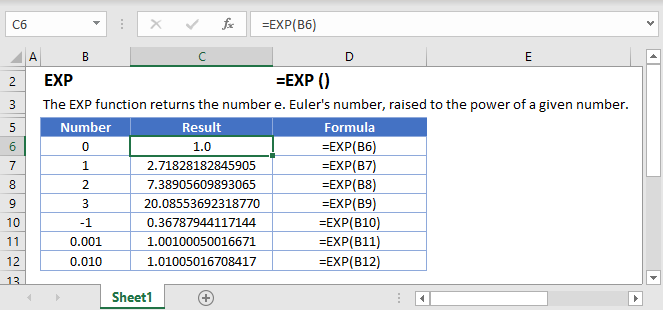## EXP Function Overview

The EXP Function Calculates the exponential value for a given number.

To use the EXP Excel Worksheet Function, select a cell and type: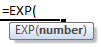(Notice how the formula inputs appear)

### EXP Function Syntax and Inputs:

`=EXP(number)`

number – A number.

AutoMacro - VBA Code Generator

### What is the EXP function?

The EXP function falls under the category of Math and Trigonometry Functions in Microsoft Excel, which includes LOG and LN. All these functions are related by the ‘natural number’, e, equal to approximately 2.71828. The inverse of EXP is the LN function, meaning that if you input a number into the the LN function and then nest that inside the EXP function, you will get your original number back:

`=LEN(EXP(3))`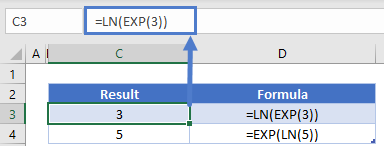EXP takes a given real number and raises e to that power.

### When would you use the EXP function?

EXP is useful when calculating things to do with growth – crop sizes, bacterial growth, animal populations, and even financial interest.

### Common Errors

#NUM – This error occurs when a number too large is input into the EXP function. The smallest value that the EXP function can take is -1×10³⁰⁷ and the largest value is 709.782712893384. But to be honest, when are you going to use numbers that extreme anyway?

For context, e⁷⁰⁹ is equal to about 8×10³⁰⁷ – that’s an 8 with 307 zeroes after it. If that were describing a number of atoms, it would be more than 3 and a half times the number of atoms in the entire universe!

#NAME – This error occurs when the input is not recognized as a number. Unfortunately, that means you cannot calculate e ͥ  or Euler’s Identity in MS Excel.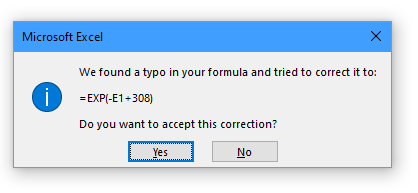Typo Error – In attempting to bypass the limits of the EXP function, you may be tempted to write your inputs in scientific notation like this: -1E308. As this number will not be recognizable to Excel, a typo error prompt will be shown.

If you accept the correction in the prompt, the formula will use the value in cell E1 as the input. If E1 is zero of blank, this will return the number 5.7903E+133.
If you do not accept the correction, Excel will tell you there is a problem with the formula.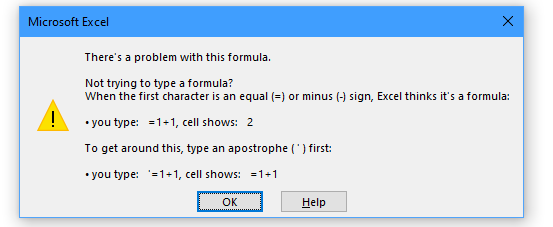### More EXP examples

In 2020 the Coronavirus spread across the planet. The world learned over a period of a couple of months what exponential growth looks like in a real-world setting. Assuming the infection rate, a, is 20%, and there was only one person initially infected with the virus, we can calculate the number of people infected on any given day. Of course, there are problems with this model but it can get us a basic idea.

The equation for working out how many people are infected at any time, t, is given by N = N₀ eᵃᵗ

If we wanted to know the number of infected on day 45 we can use Excel to calculate this:

`=C3*EXP(C4*C5)`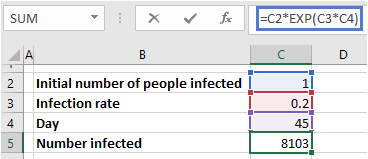So starting with only 1 person infected, and infection rate of 45 days, over 8100 people will be infected by day 45.

We can see how the infections change over time by creating a list of days and calculating each day, then graph the results.

`=\$C\$2*EXP(\$C\$3*C6)`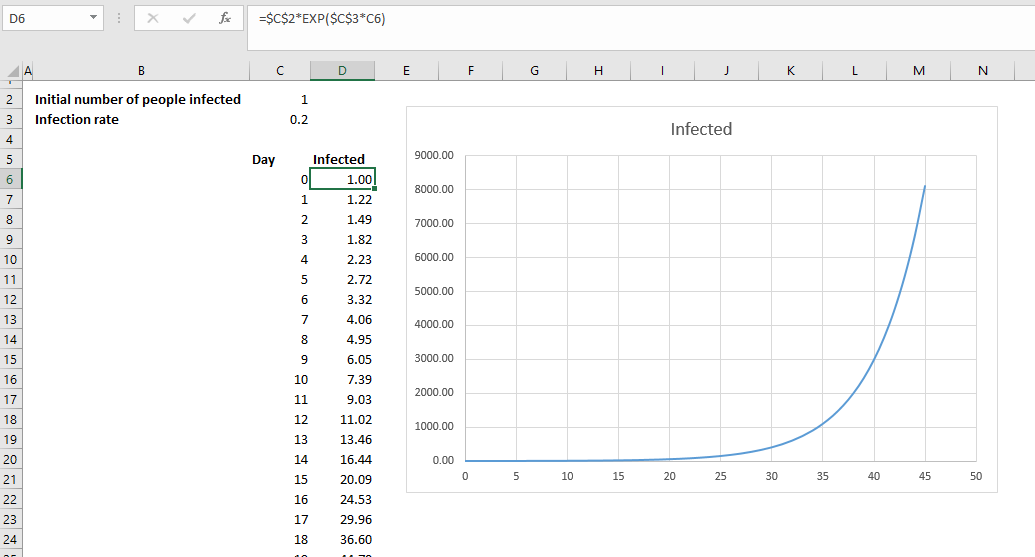This graph has similarities with real-world data, but also has some dissimilarities because we haven’t taken into account the real-world consequences of the virus like social distancing measures and fluctuating infection rates. The real-world data shows that while there were only 279 cases 45 days after the first reported case, that quickly increases to 8,000 only ten days later.

As an exercise, see if you can recreate this graph and see the effect of changing the infection rate from 20% down to 18%.

## EXP in Google Sheets

The EXP Function works exactly the same in Google Sheets as in Excel: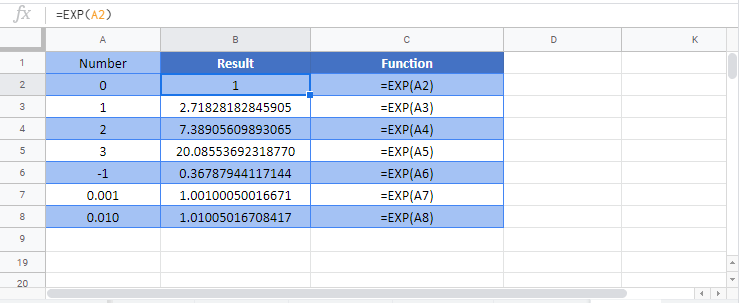## EXP Examples in VBA

You can also use the EXP function in VBA. Type: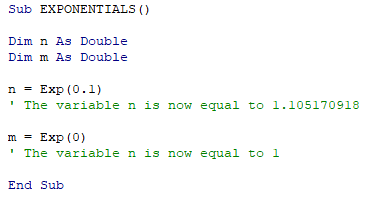## Excel Practice Worksheet

Practice Excel functions and formulas with our 100% free practice worksheets!

• Automatically Graded Exercises
• Learn Excel, Inside Excel!